Support the Monkey! Tell All your Friends and TeachersHome MonkeyNotes Printable Notes Digital Library Study Guides Study Smart Parents Tips College Planning Test Prep Fun Zone Help / FAQ How to Cite Request a New Title

8.18 Student's t-distribution

This concept has been introduced by W. S. Gosset (1876 - 1937). He adopted the pen name "student." Therefore, the distribution is known as 'students t-distribution'.

It is used to establish confidence limits and test the hypothesis when the population variance is not known and sample size is small ( < 30 ).

If a random sample x1, x2, ......., xn of n values be drawn from a normal population with mean m and standard deviation s then the mean of sample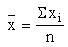Estimate of the variance : Let s2 be the estimate of the variance of the sample then s2 given by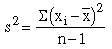( n - 1 ) as denominator in place 'n'.

( I ) The statistic 't' is defined as

t =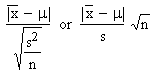Where x = sample mean, m = actual or hypotheticalmean of population, n = sample size, s = standard deviation of sample.

Where s =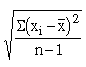Note: 't' is distributed as the student distribution with
( n - 1 ) degree of freedom (df ).

(II)1) The variable 't' distribution ranges from minus infinity to plus infinity.

2) Like standard normal distribution, it is also symmetrical and has mean zero

3) s2 of t-distribution is greater than 1, but becomes 1 as 'df' increases and thus the sample size becomes large. Thus the variance of t-distribution approaches the variance of the normal distribution as the sample size increases for n ( df ) = ¥, the t-distribution matches with the normal distribution. (observe the adjoining figure).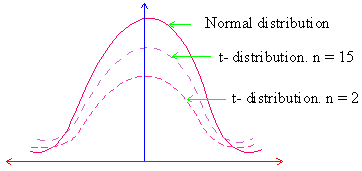Also note that the t-distribution is lower at the mean and higher at the tails than the normal distribution. The t-distribution has proportionally greater area at its tails than the normal distribution.Your browser does not support the IFRAME tag.

(III) 1) If | t | exceeds t0.05 then difference between x and m is significant at 0.05 level of significance.

2) If | t | exceeds t0.01, then difference is said to highly significant at 0.01 level of significance.

3) If | t | < t0.05 we conclude that the difference between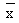and m is not significant and the sample might have been drawn from a population with mean = m i.e. the data is consistent with the hypothesis.

(IV)Fiducial limits of population mean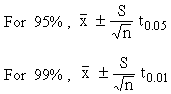Example A random sample of 16 values from a normal population is found to leave a mean of 41.5 and standard deviation of 2.795. On this basis is there any reason to reject the hypothesis that the population mean m = 43? Also find the confidence limits for m.

Solution: Here n = 16 - 1 = 15,= 41.5 , s = 2.795 and m = 43.

Now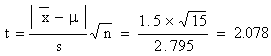From the t-table for 15 degree of freedom, the probability of t being 0.05, the value of t = 2.13. Since 2.078 < 2.13. The difference betweenand m is not significant.

Now, null hypothesis : Ho : m = 43 and

Alternative hypothesis : Ha : m ¹ 43.

Index

8.1 Population
8.2 Sample
8.3 Parameters and Statistic
8.4 Sampling Distribution
8.5 Sampling Error
8.6 Central Limit Theorem
8.7 Critical Region
8.8 Testing of Hypothesis
8.9 Errors in Tesitng of Hypothesis
8.10 Power o a Hypothesis Test
8.11 Sampling of Variables
8.12 Sampling of Attributes
8.13 Estimation
8.14 Testing the Difference Between Means
8.15 Test for Difference Between Proportions
8.16 Two Tailed and one Tailed Tests
8.17 Test of Significance for Small Samples
8.18 Students t-distribution
8.19 Distribution of 't' for Comparison of Two Samples Means Independent Samples
8.20 Testing Difference Between Mens of Two Samples Dependent Samples or Matched Paired Observations
8.21 Chi-Square
8.22 Sampling Theory of Correlation
8.23 Sampling Theory of Regression

Chapter 1Search: All Products Books Popular Music Classical Music Video DVD Toys & Games Electronics Software Tools & Hardware Outdoor Living Kitchen & Housewares Camera & Photo Cell Phones Keywords: